×#### Thank you for registering.

One of our academic counsellors will contact you within 1 working day.

Click to Chat

1800-1023-196

+91-120-4616500

CART 0

• 0

MY CART (5)

Use Coupon: CART20 and get 20% off on all online Study Material

ITEM
DETAILS
MRP
DISCOUNT
FINAL PRICE
Total Price: Rs.

There are no items in this cart.
Continue Shopping• Complete JEE Main/Advanced Course and Test Series
• OFFERED PRICE: Rs. 15,900
• View Details

```Chapter 3: Pair of Linear Equations in Two Variables Exercise – 3.9

Question: 1

A father is 3 times as old as his son. After 12 years, his age will be twice as that of his son then. Find their present ages.

Solution:

Let the present age of the father be x years. Father is 3 times as old as his son. Thus we have

x = 3y

⇒ x – 3y = 0

After 12 years, father’s age will be (x + 12) years and son’s age will be (y + 12) years. Thus using the given information, we have

x + 12 = 2(y + 12)

⇒ x + 12 = 2y + 24

⇒ x – 2y – 12 = 0

So, we have two equations

x – 3y = 0

x – 2y – 12 = 0

Here, x and y are unknowns. We have to solve the above equations for x and y.

By using cross-multiplication, we have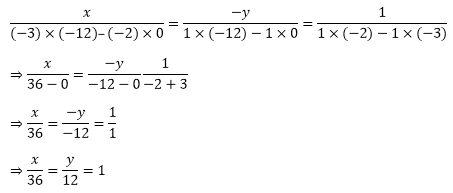x = 36, y = 12

Hence, the present age of father is 36 years and the present age of son Is 12 years.

Question: 2

Ten years later. A will be twice as old as B and five years ago, A was three times as old as B. What are the present ages of A and B.

Solution:

Let the present age of x years and the present age of B be y years.

After 10 years, A’s age will be (x +10) years and B’s age will be (y + 10) years. Thus using the given information, we have

x – 5 = 3(y – 5)

⇒ x + 10 = 2y + 20

⇒ x – 2y -10 = 0

Before 5 years, the age of A was (x – 5) years and the age of B was (y – 5) years. Thus using the given information, we have

x – 5 = 3(y - 5)

⇒ x – 5 = 3y – 15

⇒ x – 3y + 10 = 0

So, we have 2 equations

x – 2y – 10 = 0

x – 3y + 10 = 0

Here, x and y are unknowns. We have to solve the above equations for x and y. By using cross-multiplication, we have

x(−2) × 10 – (−3) × (−10) = −y1 × 10 − 1 × (−10) = 11 × (−3) − 1 × (−2)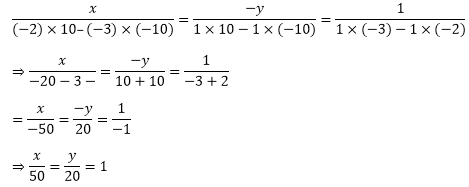⇒ x = 50, y = 20

Hence, the present age of A is 50 years and the present age if b is 20 years.

Question: 3

A is elder to B by 2 years. A’s father F is twice as old as A and B is twice as old as his sister S. If the age of the father and sister differ by 40 years, find the age of A.

Solution:

Let the present ages of A, B, F and S be x, y, z and t years respectively.

A is elder to b by 2 years. Thus, we have x = y + 2

F is twice as old as A. Thus, we have z = 2x

B is twice as old as S. Thus, we have y = 2t

The ages of F and S is differing by 40 years. Thus we have z – t = 40.

So, we have four equations

x = y + 2    …(1)

z = 2x         .…(2)

y = 2y       .…(3)

z – t = 40 …(4)

Here x, y, z and t are unknowns. We have to find the value of x. By using the third equation, the first equation becomes

x = 2t + 2

From the third equation, the first equation becomes

x = 2t + 2

From the fourth equation, we have

t = z – 40

Hence, we have

x = 2(z – 40) + 2

= 2z – 80 + 2

= 2z – 78

Using the second equation, we have

x = 2 × 2x –78 ⇒ x = 4x − 78

⇒ 4x − x = 78 ⇒ 3x = 78

⇒ x = 78/3

⇒ x = 26

Hence, the age of A is 26 years.

Question: 4

Six year hence a man’s age will be three times age of his son and three years ago he was nine times as old as his son. Find their present ages.

Solution:

Let the present age of the man be x years and the present age of his son be y years.

After 6 years, the man’s age will be (x + 6) years and son’s age will be (y + 6) years. Thus using the given information, we have

x + 6 = 3(y + 6)

x + 6 = 3y + 18

x - 3y - 12 = 0

Before 3 years, the age of the man was (x – 3) years and the age of son’s was (y — 3) years. Thus using the given information, we have

x - 3 = 9(y - 3)

x - 3 = 9y - 27

x - 9y + 24 = 0

So, we have two equations

x - 3y - 12 = 0

x - 9y + 24 = 0

Here x and y are unknowns. We have to solve the above equations for x and y. By using cross-multiplication, we have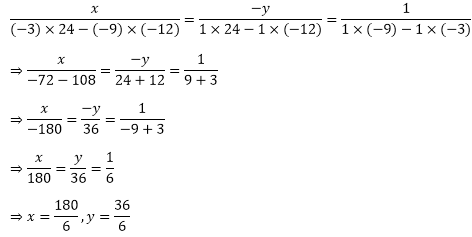⇒ x = 30, y = 6

Hence, the present age of the man is 30 years and the present age of son is 6 years.

Question: 5

Ten years ago, a father was 12 times as old as his son and 10 years hence, he will be twice as old as his son will be then. Find their present ages.

Solution:

Let the present age of father be x years and the present age of his son be y years.

After 10 years, father’s age will be (x+10) years and son’s age will be (y + 10) years. Thus using the given information, we have

x + 10 = 2(y + 10)

x - 10 = 2y + 20

x - 2y - 10 = 0

Before 10 years, the age of father was (x-10) years and the age of son was (y — 10) years. Thus using the given information, we have

x - 10 = 12(y - 10)

x - 10 = 12y — I20

x - 12y + 110 = 0

So, we have two equations

x - 2y - 10 = 0

x - 12y + 110 = 0

Here x and y are unknowns. We have to solve the above equations for x and y. By using cross-multiplication, we have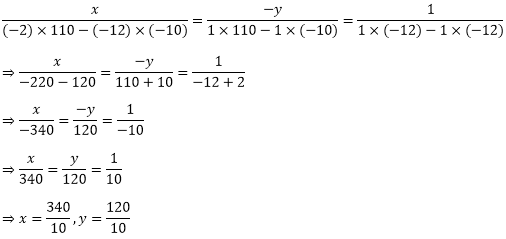⇒ x = 34, y = 12

Hence, the present age of father is 34 years and the present age of the son is 12 years.

Question: 6

The present age of father is 3 years more than three times of the age of the son. Three years hence, father’s age will be 10 years more than twice the age of the son. Determine their present age.

Solution:

Let the present age of father be x years and the present age of his son be y years.

The present age of father is three years more than three times the age of the son. Thus, we have

x = 3y + 3 x - 3y - 3 = 0

After 3 years, father’s age will be (x + 3) years and son’s age will be (y + 3) years.

Thus using the given information, we have

x + 3 = 2(y - F3) + 10

x + 3 = 4 + 6 + 10

x — 2y - 13 = 0 So.

We have two equations

x - 3y - 3 = 0

x - 2y - 13 = 0

Here x and y are unknowns. We have to solve the above equations for x and y. By using cross-multiplication, we have⇒ x = 33, y = 10

Question: 7

A father is 3 times as old as his son. In 12 years’ time, he will be twice as old as his son. Find the present ages of son and father.

Solution:

Let the present age of father be x years and the present age of his son be y years.

The present age of father is three times the age of the son. Thus, we have

x = 3y

x - 3y = 0

After 12 years, father’s age will be (x + 12) years and son’s age will be (y + 12) years.

Thus using the given information, we have

x + 12 = 2(y + 12)

x + 12 = 2y + 24

x - 2y - 12 = 0

So, we have two equations

x - 3y = 0

x - 2y - 12 = 0

Here x and y are unknowns. We have to solve the above equations for x and y. By using cross-multiplication, we have⇒ x = 36, y = 12

Hence, the present age of the father is 36 years old and the present age of son is 12 years.

Question: 8

Father’s age is three times the sum of age of his 2 children. After 5 years his age will be twice the sum of ages of two children. Find the age of father.

Solution:

Let the present age of father be x years and the present ages of his two children’s be y and z years.

The present age of father is three times the sum of the ages of the two children’s. Thus, we have

x = 3(y + z)

y + z = x/3

After 5 years, father’s age will be (x + 5) years and the children’s age will be (y + 5) and (z + 5) years. Thus using the given information, we have

x + 5 = 2{(y + 5) + (z + 5)}

x + 5 = 2(y + 5 + z + 5)

x = 2(y + z) + 20 - 5

x = 2(y + z) + 15

So, we have two equations

y + z = x/3

x = 2(y + z) + 15

Here x, y and z are unknowns. We have to find the value of x.

Substituting the value of (y + z) from the first equation in the second equation, we have

By using cross-multiplication, we have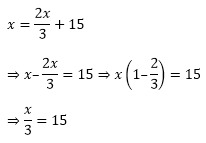⇒ x = 15 × 3

⇒ x = 45

Hence, the present age of father is 45 years.

Question: 9

Two years ago, a father was 5 times as old as his son. Two years later his age will be 8 more than 3 times the age of the son. Find the present ages of father and son.

Solution:

Let the present age of father be x years and the present age of his son be y years.

After 2 years, father’s age will be (x + 2) years and the age of son will be (y + 2) years. Thus using the given information, we have

x + 2 = 3(y +2) + 8

x + 2 = 3y + 6 + 8

x - 3y - 12 = 0

Before 2 years, the age of father was (x —2) years and the age of son was (y— 2) years. Thus using the given information, we have

x - 2 = 5(y - 2)

x - 2 = 5y - 10

x - 5y + 8 = 0

So, we have two equations

x – 3y - 12 = 0

x – 5y + 8 = 0

Here x and y are unknowns. We have to solve the above equations for x and y. By using cross-multiplication, we have⇒ x = 42, y = 10

Hence, the present age of father is 42 years and the present age of son is 10 years.

Question: 10

Five years ago, Nuri was thrice as old as Sonu. Ten years later, Nuri will be twice as old as Sonu. How old are Nuri and Sonu?

Solution:

Let the present age of Nuri be x years and the present age of Sonu be y years.

After 10 years, Nuri’s age will be(x + 10) years and the age of Sonu will be(y + 10) years. Thus using the given information, we have

x + 10 = 2(y + 10)

x + 10 = 2y + 20

x – 2y – 10 = 0

Before 5 years, the age of Nuri was (x – 5) years and the age of Sonu was (y- 5) years.

Thus using the given information, we have

x - 5 = 3(y - 5)

x – 5 = 3y - 15

x - 3y + 10 = 0

So, we have two equations

x – 2y – 10 = 0

x - 3y + 10 = 0

Here x and y are unknowns. We have to solve the above equations for x and y. By using cross-multiplication, we have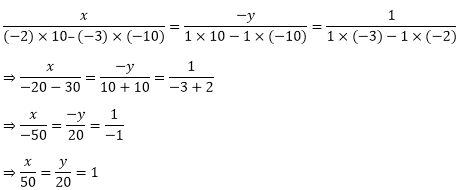⇒ x = 50, y = 20

Question: 11

The ages of two friends Ani and biju differ by 3 years. Ani’s father Dharam is twice as old as Ani and Biju as twice as old as his sister Cathy. The ages of Cathy and Dharam differ by 30 years. Find the ages of Ani and Biju.

Solution:

Let the present ages of Ani, Biju, Dharam and Cathy be x, y, z and t years respectively.

The ages of Ani and Biju differ by 3 years. Thus, we have

x – y = ±3

x = y ± 3

Dharam is twice as old as Ani. Thus, we have

z = 2x

Biju is twice as old as Cathy. Thus, we have

y = 2t

The ages of Cathy and Dharam differ by 30 years. Clearly, Dharam is older than Cathy. Thus, we have

z – t =30

So, we have two systems of simultaneous equations

(i)   x = y + 3,

z = 2x,

y = 2t,

z - t = 30

(ii) x = y - 3,

z = 2x,

y = 2t,

z – t = 30

Here x, y, z and t are unknowns. We have to find the value of x and y.

(i) By using the third equation, the first equation becomes x = 2t + 3

From the fourth equation, we have

t = z -30

Hence, we have

x = 2(z - 30) + 3

= 2z - 60 + 3

= 2z - 57

Using the second equation, we have

x = 2 × 2x − 57x = 2 × 2x − 57

x = 4x - 57

4x – x = 57

3x = 57

x = 57/3

x = 19

From the first equation, we have

x = y + 3

y = x - 3

y = 19 - 3

y = 16

Hence, the age of Ani is 19 years and the age of Biju is 16 years.

(ii) By using the third equation, the first equation becomes x= 2t -3.

From the fourth equation, we have

t = z – 30

Hence, we have

x = 2(z —30) - 3

= 2z - 60 - 3

= 2z - 63

Using the second equation, we have

x = 2 × 2x – 63

x = 4x - 63

4x – x = 63

3x = 63

x = 63/3

x = 21

From the first equation, we have

x = y - 3

y = x + 3

y = 21 + 3

y = 24

Hence, the age of Anil is 21 years and the age of Biju is 24 years.

Note that there are two possibilities.
```### Course Features

• 728 Video Lectures
• Revision Notes
• Previous Year Papers
• Mind Map
• Study Planner
• NCERT Solutions
• Discussion Forum
• Test paper with Video Solution